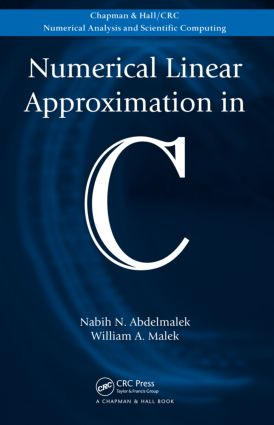Numerical Linear Approximation in C

1st Edition

Chapman and Hall/CRC

968 pages | 24 B/W Illus.

Hardback: 9781584889786
pub: 2008-05-19
Currently out of stock
\$155.00
x
eBook (VitalSource) : 9780429144622
pub: 2008-05-19
from \$28.98

FREE Standard Shipping!

Description

Illustrating the relevance of linear approximation in a variety of fields, Numerical Linear Approximation in C presents a unique collection of linear approximation algorithms that can be used to analyze, model, and compress discrete data. Developed by the lead author, the algorithms have been successfully applied to several engineering projects at the National Research Council of Canada.

Basing most of the algorithms on linear programming techniques, the book begins with an introductory section that covers applications, the simplex method, and matrices. The next three parts focus on various L1, Chebyshev, and least squares approximations, including one-sided, bounded variables, and piecewise. The final section presents the solution of underdetermined systems of consistent linear equations that are subject to different constraints on the elements of the unknown solution vector.

Except in the preliminary section, all chapters include the C functions of the algorithms, along with drivers that contain numerous test case examples and results. The accompanying CD-ROM also provides the algorithms written in C code as well as the test drivers. To use the software, it is not required to understand the theory behind each function.

Reviews

"The book represents the research career of the senior author. His love of the subject is reflected through the book. The book is a welcome addition to the literature."

—Zuhair Nashed, SIAM Review, 2009

Preliminaries and Tutorials

Applications of Linear Approximation

Applications to social sciences and economics

Applications to industry

Applications to digital images

Preliminaries

Discrete linear approximation and solution of overdetermined linear equations

Comparison between the L1, the L2, and the L norms by a practical example

Error tolerances in the calculation

Representation of vectors and matrices in C

Outliers and dealing with them

Linear Programming and the Simplex Algorithm

Notations and definitions

The simplex algorithm

The simplex tableau

The two-phase method

Duality theory in linear programming

Degeneracy in linear programming and its resolution

Linear programming and linear approximation

Stability of the solution in linear programming

Efficient Solutions of Linear Equations

Vector and matrix norms and relevant theorems

Elementary matrices

Gauss LUdecomposition with complete pivoting

Orthogonal factorization methods

Gauss–Jordan method

Rounding errors in arithmetic operations

The L1 Approximation

Linear L1 Approximation

Linear programming formulation of the problem

Description of the algorithm

The dual simplex method

Modification to the algorithm

Occurrence of degeneracy

A significant property of the L1 approximation

Triangular decomposition of the basis matrix

Arithmetic operations count

C source code

One-Sided L1 Approximation

A special problem of a general constrained one

Linear programming formulation of the problem

Description of the algorithm

C source code

L1 Approximation with Bounded Variables

A special problem of a general constrained one

Linear programming formulation of the problem

Description of the algorithm

C source code

L1 Polygonal Approximation of Plane Curves

Approaches to polygonal approximation

The L1 approximation problem

Description of the algorithm

Linear programming technique

C source code

Piecewise L1 Approximation of Plane Curves

Characteristics of the piecewise approximation

The discrete linear L1 approximation problem

Description of the algorithms

C source code

The Chebyshev Approximation

Linear Chebyshev Approximation

Linear programming formulation of the problem

Description of the algorithm

A significant property of the Chebyshev approximation

C source code

One-Sided Chebyshev Approximation

A special problem of a general constrained one

Linear programming formulation of the problem

Description of the algorithm

C source code

Chebyshev Approximation with Bounded Variables

A special problem of a general constrained one

Linear programming formulation of the problem

Description of the algorithm

C source code

Restricted Chebyshev Approximation

A special problem of general constrained algorithms

Linear programming formulation of the problem

Description of the algorithm

The triangular decomposition method

Arithmetic operations count

C source code

Strict Chebyshev Approximation

The problem as presented by Descloux

Linear programming analysis of the problem

C source code

Piecewise Chebyshev Approximation

Characteristic properties of piecewise approximation

The discrete linear Chebyshev approximation problem

Description of the algorithms

C source code

Solution of Linear Inequalities

Pattern classification problem

Solution of the system of linear inequalities Ca > 0

Linear one-sided Chebyshev approximation algorithm

Linear one-sided L1 approximation algorithm

C source code

The Least Squares Approximation

Least Squares and Pseudo-Inverses of Matrices

Least squares solution of linear equations

Factorization of matrix A

Explicit expression for the pseudo-inverse

The singular value decomposition (SVD)

Practical considerations in computing

Linear spaces and the pseudo-inverses

Multicollinearity, collinearity, or the ill-conditioning of matrix A

Principal components analysis (PCA)

Partial least squares method (PLS)

Ridge equation

C source code

Piecewise Linear Least Squares Approximation

Characteristics of the approximation

The discrete linear least squares approximation problem

Description of the algorithms

Updating and downdating techniques

C source code

Solution of Ill-Posed Linear Systems

Solution of ill-posed linear systems

Estimation of the free parameter

Description of the new algorithm

Optimum value of the rank

Use of linear programming techniques

C source code

Solution of Underdetermined Systems Of Linear Equations

L1 Solution of Underdetermined Linear Equations

Linear programming formulation of the problem

Description of the algorithm

C source code

Bounded and L1 Bounded Solutions of Underdetermined Linear Equations

Linear programming formulation of the two problems

Description of the algorithms

C source code

Chebyshev Solution of Underdetermined Linear Equations

The linear programming problem

Description of the algorithm

C source code

Bounded Least Squares Solution of Underdetermined Linear Equations

Quadratic programming formulation of the problems

Solution of problem (E0)

Solution of problem (E)

C source code

Appendix A: References

Appendix B: Main Program

Appendix C: Constants, Types, and Function Prototypes

Appendix D: Utilities and Common Functions

Index

An Introduction appears at the beginning of each chapter.